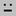# 7L 3s

Jump to: navigation, search

# 7+3 - "unfair mosh" - "neutral third scales"

"7L 3s" refers to the structure of moment of symmetry scales built from a 10-tone chain of neutral thirds (assuming a period of an octave):

L s L L L s L L s L

Graham Breed has a page on his website dedicated to 7+3 scales. He proposes calling the large step "t" for "tone", lowercase because the large step is a narrow neutral tone, and the small step "q" for "quartertone", because the small step is often close to a quartertone. (Note that the small step is not a quartertone in every instance of 7+3, so do not take that "q" literally.) Thus we have:

t q t t t q t t q t

# Interval ranges

The generator (g) will fall between 343 cents (2\7 - two degrees of 7edo and 360 cents (3\10 - three degrees of 10edo) - the perceptual zone of a "neutral third".

2g, then, will fall between 686 cents (4\7) and 720 cents (3\5) - the perceptual zone of a "perfect fifth".

The "large step" will fall between 171 cents (1\7) and 120 cents (1\10) - the perceptual zone of "neutral second".

The "small step" will fall between 0 cents and 120 cents, sometimes sounding like a minor second, and sometimes sounding like a quartertone or smaller microtone.

The most frequent interval, then is the neutral third (and its inversion, the neutral sixth), followed by the perfect fourth and fifth. Thus, 7+3 combines the familiar sound of perfect fifths and fourths with the unfamiliar sounds of neutral intervals. They are compatible with Arabic and Turkish scales, but not with traditional Western ones.

# A continuum of compatible edos

The generator range reflects two extremes: one where L=s (3\10), and another where s=0 (2\7). Between these extremes, there is an infinite continuum of possible generator sizes. By taking freshman sums of the two edges (adding the numerators, then adding the denominators), we can fill in this continuum with compatible edos, increasing in number of tones as we continue filling in the in-betweens. Thus, the smallest in-between edo would be (3+2)\(10+7) = 5\17 -- five degrees of 17edo:

Generator (fraction of octave) Generator (cents) Comments
3\10 360
17\57 357.895
14\47 357.45
11\37 356.76
8\27 355.56 Optimum rank range (L/s=3/2)
355.11
21\71 354.93
34\115 354.78 Golden neutral thirds
13\44 354.55
354.19
5\17 352.94 Boundary of propriety: generators larger than this are proper
12\41 351.22
31\106 350.94 No-5's neutral thirds (observing 81/80) is around here
19\65 350.77
350.64 L/s = e
7\24 350
349.72 L/s = pi
16\55 349.09
9\31 348.39 Mohajira (tempering out 81/80) is around here
11\38 347.37
13\45 346.67
2\7 342.86

The scale produced by stacks of 5\17 is the 17edo neutral scale. Between 11/38 and 16/55, with 9/31 in between, is the mohajira/mohaha/mohoho range, where mohaha and mohoho use the MOS as the chromatic scale of a chromatic pair.

Other compatible edos include: 37edo, 27edo, 44edo, 41edo, 24edo, 31edo.

You can also build this scale by stacking neutral thirds that are not members of edos -- for instance, frequency ratios 11:9, 49:40, 27:22, 16:13 -- or the square root of 3:2 (a bisected just perfect fifth).

# 7-note subsets

If you stop the chain at 7 tones, you have a heptatonic scale of the form 3L 4s:

L s s L s L s

The large steps here consist of t+s of the 10-tone system, and the small step is the same as t. Graham proposes calling the large step here T for "tone," uppercase because it is a wider tone than t. Thus, we have:

T t t T t T t

This scale (and its rotations) is not the only possible heptatonic scale. Graham also gives us:

T t t T t t T

which is not a complete moment of symmetry scale in itself, but a subset of one.

# Tetrachordal structure

Due to the frequency of perfect fourths and fifths in this scale, it can also be analyzed as a tetrachordal scale. The perfect fourth can be traversed by 3 t's and a q, or 2 t's and a T.

I (- Andrew Heathwaite) offer "a" to refer to a step of 2t (for "augmented second")

Thus, the possible tetrachords are:

T t t

t T t

t t T

a q t

a t q

t a q

t q a

q a t

q t a